Data Interpretation MCQ -4

# Data Interpretation MCQ -4

Test Description

## 20 Questions MCQ Test Quantitative Techniques for CLAT | Data Interpretation MCQ -4

Data Interpretation MCQ -4 for Banking Exams 2022 is part of Quantitative Techniques for CLAT preparation. The Data Interpretation MCQ -4 questions and answers have been prepared according to the Banking Exams exam syllabus.The Data Interpretation MCQ -4 MCQs are made for Banking Exams 2022 Exam. Find important definitions, questions, notes, meanings, examples, exercises, MCQs and online tests for Data Interpretation MCQ -4 below.
Solutions of Data Interpretation MCQ -4 questions in English are available as part of our Quantitative Techniques for CLAT for Banking Exams & Data Interpretation MCQ -4 solutions in Hindi for Quantitative Techniques for CLAT course. Download more important topics, notes, lectures and mock test series for Banking Exams Exam by signing up for free. Attempt Data Interpretation MCQ -4 | 20 questions in 15 minutes | Mock test for Banking Exams preparation | Free important questions MCQ to study Quantitative Techniques for CLAT for Banking Exams Exam | Download free PDF with solutions
 1 Crore+ students have signed up on EduRev. Have you?
Data Interpretation MCQ -4 - Question 1

### Study the following graph carefully to answer the given questions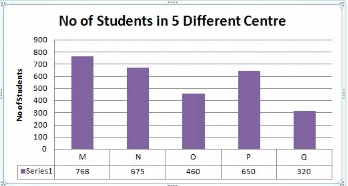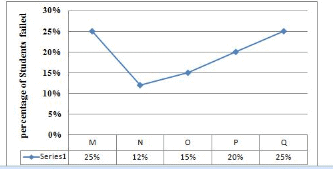If the ratio of number of boys & girls are in the ratio 3:2 in N and P, find the average number of boys in N and P together ?

Detailed Solution for Data Interpretation MCQ -4 - Question 1

N:675 =>Boys = 675*3/5 = 405
P:650 =>Boys = 650*3/5 = 390
Avg = 405+390/2 = 397.5

Data Interpretation MCQ -4 - Question 2

### Study the following graph carefully to answer the given questionsFind the total no of failed students in M, O and Q together ?

Detailed Solution for Data Interpretation MCQ -4 - Question 2

M =768*25%= 192
O= 460*15% = 69
Q = 320*25% = 80
Total = 341

Data Interpretation MCQ -4 - Question 3

### Study the following graph carefully to answer the given questionsWhat will be the ratio of number of boys passed in N to number of girls failed in N, if the number of girls and boys in the ratio 16:11 ?

Detailed Solution for Data Interpretation MCQ -4 - Question 3

675 => 400:275 => 16:11 = G:B
G:400*12% =48
B:275*88% =242
242:48 = 121: 24

Data Interpretation MCQ -4 - Question 4

Study the following graph carefully to answer the given questionsFind the difference between the number of passed students in Q to number of failed students in M ?

Detailed Solution for Data Interpretation MCQ -4 - Question 4

Q passed => 75% of 320 = 240
M failed => 192
Difference = 240-192 = 48

Data Interpretation MCQ -4 - Question 5

Study the following graph carefully to answer the given questionsWhich centre has the second least number of failed student ?

Detailed Solution for Data Interpretation MCQ -4 - Question 5

M=192
N=81
O=69
P=130
Q=80

Data Interpretation MCQ -4 - Question 6

Study the following table carefully to answer the given questions

Classification of 50 Students based on the marks obtained by them in Quants & Reasoning in Prelim exam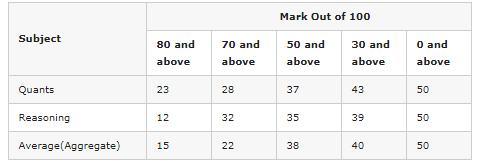The number of students scoring less than 30% marks in aggregate is?

Detailed Solution for Data Interpretation MCQ -4 - Question 6

We have 30% of 100 = (30/100)x100 = 30
Therefore Required number
= Number of students scoring less than 30 marks in aggreagate
= 50 – Number of students scoring 30 and above marks in aggregate
= 50 – 40 = 10.

Data Interpretation MCQ -4 - Question 7

Study the following table carefully to answer the given questions

Classification of 50 Students based on the marks obtained by them in Quants & Reasoning in Prelim examIf it is known that at least 20 students were eligible for main exam, then the minimum qualifying marks in Reasoning for eligibility to attend the main exam(Top 20 students only can attend main exam )?

Detailed Solution for Data Interpretation MCQ -4 - Question 7

12 students secured above 80 then remaining 8 student will scored in the range 70-80

Data Interpretation MCQ -4 - Question 8

Study the following table carefully to answer the given questions

Classification of 50 Students based on the marks obtained by them in Quants & Reasoning in Prelim examThe percentage of number of students scored 70% marks in Quants is what percentage of number of students scored 30% marks in aggregate ?

Detailed Solution for Data Interpretation MCQ -4 - Question 8

Number of students scored 70% marks or 70 in Quants  = 28
Number of students scored 30% marks or 30 in aggregate= 40
% = 28/40 * 100 = 70%

Data Interpretation MCQ -4 - Question 9

Study the following table carefully to answer the given questions

Classification of 50 Students based on the marks obtained by them in Quants & Reasoning in Prelim examIf at least 50% marks in aggregate are required for interview, how many students will be eligible to attend interview?

Detailed Solution for Data Interpretation MCQ -4 - Question 9

50% of 100 = 50*100/100 = 50
No of students scored 50 aggregate = 38

Data Interpretation MCQ -4 - Question 10

Study the following table carefully to answer the given questions

Classification of 50 Students based on the marks obtained by them in Quants & Reasoning in Prelim examWhat is the different between the number of students passed with 80 as cut-off marks in Quants and 30 as cut-off marks in aggregate together to no of students passed with 70 as cut-off marks in aggregate and 50 as cut off in Reasoning?

Detailed Solution for Data Interpretation MCQ -4 - Question 10

Difference = (23+40 ) – ( 22+35) =6

Data Interpretation MCQ -4 - Question 11

(Q.no: 11-15). Refer to the following Line Graph and answer the given questions.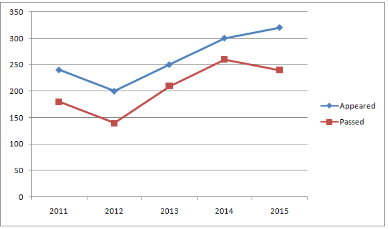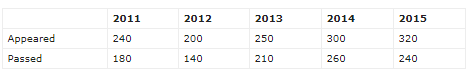The number of students who could pass the exam in the year 2016 were 10% more than that in the previous year. Only 75% of those who appeared in the year 2016 could pass the exam that year. How many students appeared for the exam in the year 2016?

Detailed Solution for Data Interpretation MCQ -4 - Question 11

Number of students who could pass the exam in the year 2016 = 110% of 240 = 264
75% of x = 264
x = 264 * 100/75 = 352

Data Interpretation MCQ -4 - Question 12

(Q.no: 11-15). Refer to the following Line Graph and answer the given questions.Number of students who had passed the exam in the year 2012 are what percent less than that in the year 2013?

Detailed Solution for Data Interpretation MCQ -4 - Question 12

Required % = (70/210) * 100 = 100/3 %

Data Interpretation MCQ -4 - Question 13

(Q.no: 11-15). Refer to the following Line Graph and answer the given questions.What percent of students who appeared for the exam in the year 2011 could pass the exam?

Detailed Solution for Data Interpretation MCQ -4 - Question 13

% = 180/240 * 100 = 75%

Data Interpretation MCQ -4 - Question 14

(Q.no: 11-15). Refer to the following Line Graph and answer the given questions.What is the respective ratio of total number of students who appeared for the exam in the years 2014 and 2015 together and the total number of students who could pass the exam in the same years together?

Detailed Solution for Data Interpretation MCQ -4 - Question 14

Total number of appeared candidates in the years 2014 and 2015 together = 620
Total number of passed candidates in the years 2014 and 2015 together = 520
= 620 : 500
= 31 : 25

Data Interpretation MCQ -4 - Question 15

(Q.no: 11-15). Refer to the following Line Graph and answer the given questions.What is the average number of students who passed for the exam in all the years together?

Detailed Solution for Data Interpretation MCQ -4 - Question 15

Average number of students who passed in all the years together =[180 + 140 + 210 + 260 +240]/5 = 206

Data Interpretation MCQ -4 - Question 16

(Q.no: 16-20). Refer to the following table and answer the given questions.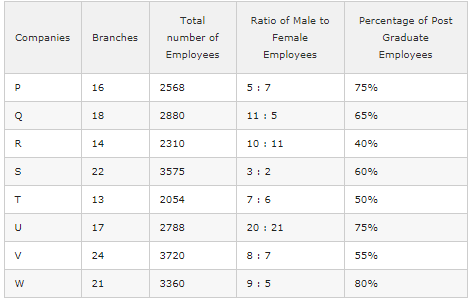If the number of male post graduate employees in company W is 1488, what percent of the female employees in that particular company are post graduate?

Detailed Solution for Data Interpretation MCQ -4 - Question 16

Number of Females in Company W = 3360 *(5/14) = 1200
Number of Post graduate employees in Company W = 3360 *(80/100) = 2688
Number of female post graduate employees in Company W = 2688 – 1488 = 1200
Required Percentage = (1200 * 100)/1200 = 100%

Data Interpretation MCQ -4 - Question 17

(Q.no: 16-20). Refer to the following table and answer the given questions.In which of the given companies is the percentage of women employees with respect to the total number of employees(both males and females) in that company the third lowest?

Detailed Solution for Data Interpretation MCQ -4 - Question 17

Company P : ([7/12 * (2568)] / 2568)*100 = 58.33%
Company Q : ([5/16 * (2880)] / 2880)*100 = 31.25%
Company R : ([11/21 * (2310)] / 2310)*100 = 52.38%
Company S : ([2/5 * (3575)] / 3575)*100 = 40%
Company T : ([6/13 * (2054)] / 2054)*100 = 46.15%
Company U : ([21/41 * (2788)] / 2788)*100 = 51.21%
Company V : ([7/15 * (3720)] / 3720)*100 = 46.66%
Company W : ([5/14 * (3360)] / 3360)*100 = 35.71%

Data Interpretation MCQ -4 - Question 18

(Q.no: 16-20). Refer to the following table and answer the given questions.What is the ratio of the total number of male employees in companies Q and W together to the total number of female employees in Companies R and S together?

Detailed Solution for Data Interpretation MCQ -4 - Question 18

= [2880 * (11/16)/ 2310 * (11/21) + 3360 * (9/14)/ 3575 * (2/5)] = (1980 + 2160)/(1210 + 1430) = 414/264 = 69:44

Data Interpretation MCQ -4 - Question 19

(Q.no: 16-20). Refer to the following table and answer the given questions.What is the sum of the average number of post graduate employees in companies P, Q and S together and the average number of post graduate employees in companies U, V and W?

Detailed Solution for Data Interpretation MCQ -4 - Question 19

Average number of post graduate employees in companies P, Q and S = (1926 + 1872 + 2145)/3 = 5943/3 = 1981
Average number of post graduate employees in companies U, V and W = (2091 + 2046 + 2688)/3 = 6875/3 = 2275
Difference = 2275 + 1981 = 4256

Data Interpretation MCQ -4 - Question 20

(Q.no: 16-20). Refer to the following table and answer the given questions.Which of the given companies has the second highest number of average employees per office?

Detailed Solution for Data Interpretation MCQ -4 - Question 20

Company P – 2568/16 = 160.5 ; Company Q – 2880/18 = 160
Company R – 2310/14 = 165 ; Company S – 3575/22 = 162.5
Company T – 2054/13 = 158 ; Company U – 2788/17 = 164
Company V – 3720/24 = 155 ; Company W – 3360/21 = 160

## Quantitative Techniques for CLAT

56 videos|35 docs|91 tests
 Use Code STAYHOME200 and get INR 200 additional OFF Use Coupon Code
Information about Data Interpretation MCQ -4 Page
In this test you can find the Exam questions for Data Interpretation MCQ -4 solved & explained in the simplest way possible. Besides giving Questions and answers for Data Interpretation MCQ -4, EduRev gives you an ample number of Online tests for practice

## Quantitative Techniques for CLAT

56 videos|35 docs|91 tests